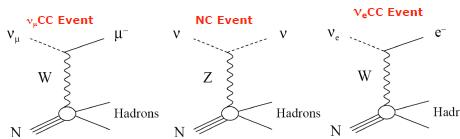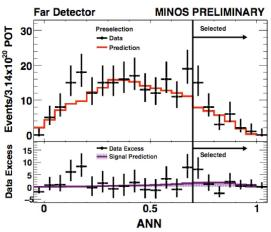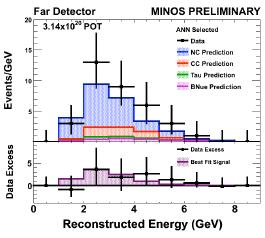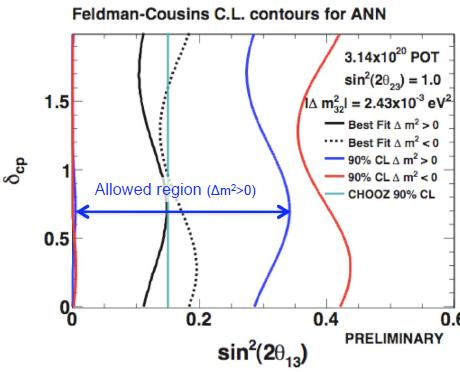## Milind Diwan: recent MINOS resultsApril 8, 2009

Posted by dorigo in news, physics, science.
Tags: , , , ,

I offer below another piece of the notes I took at the NEUTEL09 conference in Venice last month. For the slides of the talk reported here, see the conference site.Milind’s presentation concentrated on results of muon-neutrino to electron-neutrino conversions. Minos is a “Main-Injecor Neutrino Oscillation Search”. It is a long-baseline experiment: the beam from the Main injector, Fermilab’s high-intensity source of protons feeding the Tevatron accelerator, can be sent from Batavia (IL) 735km away to the Soudan mine in Minnesota. There are actually two detectors, a near and a far detector: this is the unique feature of MINOS. The spectra collected at the two sites are compared to measure muon neutrino disappearance and appearance. The near detector is 1km away from the target.

The beam is a horn-focused muon-neutrino one. Horns are parabolic-shaped magnets. 120 GeV protons originate pions, which are focused by these structures; negative ones are defocused, and the beam contains predominantly muon neutrinos from the decay of these pions. The accelerator provides 10-microsecond pulses every 2.2 seconds, with$3.3 \times 10^{13}$ protons per pulse. 95% of the resulting neutrino flux is$\nu_\mu$, 4% are$\bar \nu_\mu$.

Besides the presence of two detectors in line, another unique feature of the Fermilab beam is the possibility to move the target in and out, shifting the spectrum of neutrinos that come out, because the focal point of the horns changes. Two positions of the target are used, corresponding to two beam configurations. In the high-energy configuration one can get a beam centered at an energy of 8 GeV or so, while the low-energy configuration is centered at 3 GeV. Most of the time Minos runs with the 3 GeV beam.

Detectors are a kiloton-worth of steel and scintillator planes in the near detector, and 5.4-kT in the far detector. Scintillator strips are 1 cm thick, 4.1 cm wide, and their Moliere radius is of 3.7cm. A 1-GeV muon crosses 27 planes. The iron in the detectors is magnetized with a 1 Tesla magnetic field.

Minos event topologies include CC-like and NC-like events. A charged-current (CC) event gives a muon plus hadrons: a long charged track from the muon, which is easy to find. A neutral current (NC) event will make a splash and it is diffuse, since all one sees is the signal from the disgregation of the target nucleus; an electron CC event will leave a dense, short shower, with a typical electromagnetic shower profile. The three processes giving rise to the observed signatures are described by the Feynman diagrams below.The analysis challenge is to put together a selection algorithm capable of rejecting backgrounds and select CC$\nu_e$ events. Fluxes are measured in the near detector, and they allow to predict what can be found in the far detector. This minimizes the dependence on MC, because there are too many factors that may cause fluctuations in the real data, and the simulation cannot possibly handle them all. So they carry out a blind analysis. They check background estimates with independent samples: this serves the purpose of avoiding to bias oneself with what one should observe. They generate many simulated samples not containing an oscillation signal, to check all analysis procedures.

Basic cuts are applied on their data sample to ensure data quality. Fiducial volume cuts provide rejection to cosmic ray backgrounds. Simple cuts lead to a S/N ratio of 1:12. By “signal” one means the appearance of electron neutrinos.$\nu_e$ events are selected with artificial Neural Networks, which use the properties of the shower, the lateral shower spread, etcetera. These can discriminate NC interactions from electron-neutrino-induced CC interactions. After the application of the algorithm, the S/N ratio is 1/4. At this stage, one important remaining source of background is due to muon-neutrino CC backgrounds which can be mistaken from electron-neutrino ones when the muon is not seen in the detector.

They can select$\nu_e$ events with a “library event matching” (LEM). This matches the data event with a shower library, reconstructing the fraction of the best 50 matches which are electron-neutrino events. This is more or less like an evolved “nearest-neighbor” algorithm. As a result, they get a better separation. However, according to the speaker this method is not ready yet, since they still need to understand its details better.

[As I was taking these notes, I observed that data and Monte Carlo simulation do not match well in the low-ANN output region. The speaker claims tha the fraction of events in the tail of the Monte Carlo distribution can be modeled only with some approximation, but that they do not need to model that region too well for their result. However, it looks as if the discrepancy between data and MC not well understood. Please refer to the graph shown below, which shows the NN output in data and simulation at a preselection level.]Back to the presentation. To obtain a result, the calculation they performis simple: how many events are expected in the far detector ? The ratio of far to near flux is known, 1.3E-6. This includes all geometrical factors. For this analysis they have 3E20 protons on target. They expect 27 events for the ANN selection, and 22 for the LEM analysis.

They need to separate backgrounds in NC and CC, so they do a trick: they take data in two different beam configurations, then they look at the spectrum in the near detector, where they expect muon-type events to be rejected much more easily because they are more deflected. From this they can separate the two contributions.

Their final result for the predicted number of electron-induced CC events is 27+-5 (stat)+-2 (syst).

A second check on the background calculation consists in removing the muon in tagged CC events, and use these for two different calculations. One is an independent background calculation: they can add a simulated electron to the event raw data after removing the muon. This checks whether the signal is modeled correctly. From these studies they conclude that the signal is modeled well.The results show that there is indeed a small signal: they observe 35 events, when they expect 27, in the high-NN output region, as shown in the figure above. For the other method, LEM, results are consistent. The energy spectrum of the selected events is shown in the graph below.With the observation of this small excess (which is compatible with predictions), a confidence level is set in the plane of the two parameters$\sin^2 2 \theta_{13}$ versus$\delta_{cp}$, at 90%. It goes up to 0.35, with a small oscillation dependent on the value of$\delta$. You can see it in the figure on the right below.The speaker claims that if the excess they are observing disappears with further accumulated data, they will be able to reach below the existing bound.

The other result of MINOS is a$\delta M^2$ result from disappearance studies. The signal amounts to several hundred events of deficit. They can put a limit on an empirical parameter which determines what fraction of the initial flux has gone into sterile neutrinos. They have 6.6E20 protons on target now taken. The fraction of sterile
neutrinos is less than 0.68 at 90%CL.

## Neutrino Telescopes XIIIMarch 8, 2009

Posted by dorigo in astronomy, cosmology, news, personal, physics, science, travel.
Tags: , , , ,

The conference “Neutrino Telescopes” has arrived at its XIII edition. It is a very nicely organized workshop, held in Venice every year towards the end of the winter or the start of the spring. For me it is especially pleasing to attend, since the venue, Palazzo Franchetti (see picture below) is located at a ten minute walk from my home: a nice change from my usual hour-long commute with Padova by train.This year the conference will start on Tuesday, March 10th, and will last until Friday. I will be blogging from there, hopefully describing some new results heard in the several interesting talks that have been scheduled. Let me mention only a few of the talks, pasted from the program:

• D. Meloni (University of Roma Tre)
CP Violation in Neutrino Physics and New Physics
• K. Hoffman (University of Maryland)
AMANDA and IceCube Results
• S. Enomoto (Tohoku University)
Using Neutrinos to study the Earth
• D.F. Cowen (Penn State University)
The Physics Potential of IceCube’s Deep Core Sub-Detector
• S. Katsanevas (Université de Paris 7)
Toward a European Megaton Neutrino Observatory
• E. Lisi (INFN, Bari)
Core-Collapse Supernovae: When Neutrinos get to know Each
Other
• G. Altarelli (University of Roma Tre & CERN)
Recent Developments of Models of Neutrino Mixing
• M. Mezzetto (INFN, Padova)
Next Challenge in Neutrino Physics: the θ13 Angle
• M. Cirelli (IPhT-CEA, Saclay)
PAMELA, ATIC and Dark Matter

The conference will close with a round table: here are the participants:

Chair: N. Cabibbo (University of Roma “La Sapienza”)
B. Barish (CALTECH)
L. Maiani (CNR)
V.A. Matveev (INR of RAS, Moscow)
H. Minakata (Tokyo Metropolitan University)
P.J. Oddone (FNAL)
R. Petronzio (INFN, Roma)
C. Rubbia (CERN)
M. Spiro (CEA, Saclay)
A. Suzuki (KEK)

Needless to say, I look forward to a very interesting week!

## Vernon Barger: perspectives on neutrino physicsMay 22, 2008

Posted by dorigo in cosmology, news, physics, science.
Tags: , , ,

Yesterday morning Barger gave the first talk at PPC 2008, discussing the status and the perspectives of research in physics and cosmology with neutrinos. I offer my notes of his talk below.

Neutrinos mix among each other and have mass. There is a matrix connecting flavor and mass eigenstates, and the matrix is parametrized by three angles and a phase. To these can be addded two majorana phases for the neutrinoless double beta decay:$\phi_2$ and$\phi_3$. Practically speaking these are unmeasurable.

What do we know about these parameters ? We are at the 10th year anniversary of the groundbreaking discovery of SuperKamiokande. This was then confirmed by other neutrino experiments: MACRO, K2K, MINOS. The new result by MINOS has a 6.5 sigma significance in the low energy region. This allows to measure the mass difference precisely. It is found that$(\Delta m^2)_{12} = 2.4 \times 10^{-3}$ eV squared at$\sin^2 \theta_{23}=1.00$. The mixing angle is maximal, but we do not really know because there is a substantial error on it.

Solar neutrino oscillations are a mystery that existed for years. The flux of solar neutrinos was calculated by Bahcall, and there was a deficit. The deficit has an energy structure, as measured by the Gallium, Chlorine, and SuperK and SNO experiments by looking in neutrinos coming from different reactions -because of the different energy thresholds of the detectors: pp interactions, Beryllium 7, and Boron 8 neutrinos.
The interpretation of the data, which evolved over time, is now that the solar mixing angle is quite large, and what happens is that the high energy neutrinos sent here are created in the center of the sun, but they make a transition in matter, an adiabatic transition to a state$\nu_2$ which travels to the earth. This happens to the matter-dominated higher energy neutrinos. The vacuum dominated ones at lower energy have a different phenomenology.

There is a new result from Borexino, they measured neutrinos from the Beryllium line, and they reported a result consistent with others. Borexino is going to measure with 2% accuracy the deficit, and if KamLand has enough purity they can also go down to about 5% accuracy.

Kamland data provides a beautiful confirmation of the solution of the solar neutrino problem: solar parameters are measured precisely.$M^2_{21}$ vs$\tan^2 \theta_{12}$. The survival probability as a function of distance versus neutrino energy has a beautiful oscilation. The result only assumes CPT invariance. The angle$\theta_{12}$ is 34.4° with 1° accuracy, and$(\Delta_m^2)_{12} = 7.6 \times 10^{-5}$ eV squared.

There is one remaining angle to determine,$\theta_{13}$. From reactor experiments you expect that the probability for electron neutrino disappearance is zero in short baseline experiments. Chooz had a limit at$\theta_{13} < 11.5$ degrees. There are experiments that have sensitivities on$\sin^2 \theta_{13}$ of 0.02 (Double CHooz, DB, Reno). The angle is crucial because iti s a gateway to CP violation. If the angle is zero CP violation is not accessible in this sector.

What do we expect theoretically on$\theta_{13}$ ? There are a number of models to interpret the data. Predictions cluster around 0.01. Half of the models will be tested with the predicted accuracies of planned experiments.

There is a model called tri-bimaximal mixing: a composition analogous to the quark model basis of neutral pions, eta and eta’ mesons. An intriguing matrix: it could be a new symmetry of nature, possibly softly broken with a slightly non-zero value of the angle$\theta_x$. Or, it could well be an accident.

So, we need to find what$\theta_{13}$ is. It is zero in the Tri-binaximal mixing. We then need to measure hte mass hierarchy: is it normal ( the third state much heavier than the other two) or inverted (the lightest much lighter than the others) ? Also, is CP violated ?

Neutrinoless double-beta decay can tell us if the neutrinos are Majorana particles. In the inverted hierarchy, you measure the average mass versus the sum. There is a lot of experimental activity going on here.

Cosmic microwave has put a limit on sum of masses at 0.6 eV. By doing 21cm tomography one can measure neutrino masses with precision of 0.007 eV. If this is realizable, it could individually measure the masses of these fascinating particles.

Barger then mentioned the idea of mapping the universe with neutrinos: the idea is that active galactic nuclei (AGN) produce hadronic interactions with pions decaying to neutrinos, and there is a whole range of experiments looking at this. You could study the neutrinos coming from AGN and their flavor composition.
Another late-breaking development is that Auger has shown a correlation of ultra high-energy cosmic rays with AGNs in the sky: the cosmic rays seem to arrive directly from the nuclei of active galaxies. Auger found a higher correlation with sources at 100 Mpc, but falling off at higher distances. Cosmic rays are already probing the AGN, and this is very good news for neutrino experiments.

Then he discussed neutrinos from the sun: annihilation of weakly interacting massive particles (WIMPS), dark matter candidates, can give neutrinos from WW, ZZ, ttbar production. The idea is that the sun captured these particles gravitationally during its history, and the particles annihilate in the center of the sun, letting neutrinos escape with high energy. The ICECUBE discovery potential is high if the spin-dependent cross section for WIMP interaction in the sun is sufficiently high.

In conclusion, we have a rich panorama of experiments that all make use of neutrinos as probes of exotic phenomena as well as processes which we have to measure better to gain understanding of fundamental physics as well as gather information about the universe.